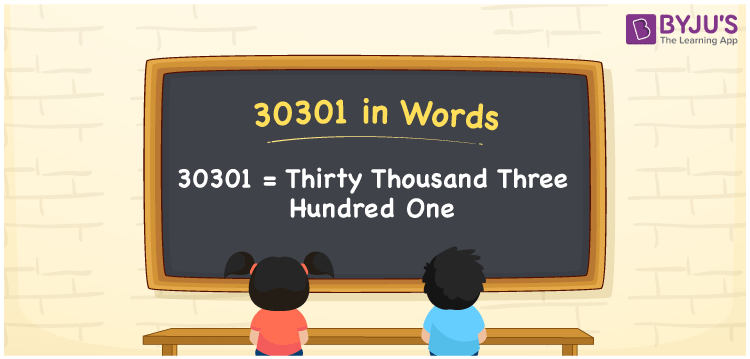# 30301 in Words

We can write 30301 in words as Thirty thousand three hundred one. If you found a journal with 30301 entries, then you can say, “I found a journal with Thirty thousand three hundred one entries”. In this article, you will get the easiest method of writing the cardinal number 30301 in words.

 30301 in words Thirty thousand three hundred one Thirty thousand three hundred one in Numbers 30301

## 30301 in English words

Generally, we write numbers in words using the English alphabet. So, we spell 30301 in English as “Thirty thousand three hundred one”.## How to Write 30301 in Words?

Below is the five-column table that represents the place values for all the digits of the number 30301.

 Ten thousands Thousands Hundreds Tens Ones 3 0 3 0 1

Here, ones = 1, tens = 0, hundreds = 3, thousands = 0, ten thousands = 3

We can expand these digits with respect to their place values as:

3 × Ten thousand + 0 × Thousand + 3 × Hundred + 0 × Ten + 1 × One

= 3 × 10000 + 0 × 1000 + 3 × 100 + 0 × 10 + 1 × 1

= 30000 + 300 + 1

= Thirty thousand + Three hundred + One

= Thirty thousand three hundred one

Therefore, 30301 in words = Thirty thousand three hundred one.

30301 is a natural number that is the successor of 30300 and the predecessor of 30302.

30301 in words – Thirty thousand three hundred one

Is 30301 an even number? – No

Is 30301 an odd number? – Yes

Is 30301 a perfect square number? – No

Is 30301 a perfect cube number? – No

Is 30301 a prime number? – No

Is 30301 a composite number? – Yes

## Frequently Asked Questions on 30301 in Words

Q1

### What is the number name of 30301?

The number name of 30301 is Thirty thousand three hundred one.
Q2

### How to write Rs. 30301 in words on a cheque?

On a cheque, we can express an amount of 30301 in words as “Thirty thousand three hundred one rupees only”.
Q3

### Write the value of 30301 + 36299 in words.

30301 + 36299 = 66600 Thus, the value of 30301 + 36299, i.e., 66600 can be written in words as Sixty-six thousand six hundred.